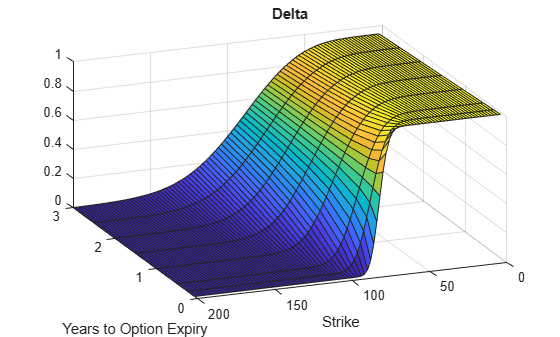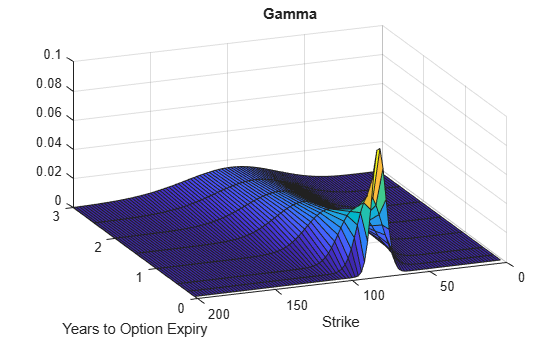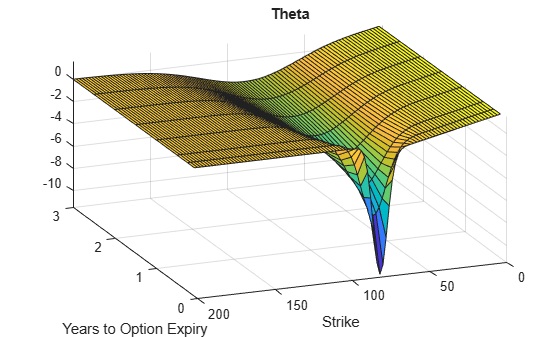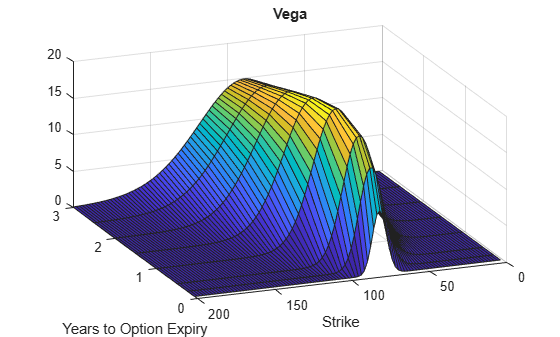Documentation

# optSensByHestonFFT

Option price and sensitivities by Heston model using FFT and FRFT

## Syntax

``[PriceSens,StrikeOut] = optSensByHestonFFT(Rate,AssetPrice,Settle,Maturity,OptSpec,Strike,V0,ThetaV,Kappa,SigmaV,RhoSV)``
``[PriceSens,StrikeOut] = optSensByHestonFFT(___,Name,Value)``

## Description

example

````[PriceSens,StrikeOut] = optSensByHestonFFT(Rate,AssetPrice,Settle,Maturity,OptSpec,Strike,V0,ThetaV,Kappa,SigmaV,RhoSV)` computes vanilla European option price and sensitivities by Heston model, using Carr-Madan FFT and Chourdakis FRFT methods.```

example

````[PriceSens,StrikeOut] = optSensByHestonFFT(___,Name,Value)` adds optional name-value pair arguments. ```

## Examples

collapse all

Use `optSensByHestonFFT` to calibrate the FFT strike grid for sensitivities, compute option sensitivities, and plot option sensitivity surfaces.

Define Option Variables and Heston Model Parameters

```AssetPrice = 80; Rate = 0.03; DividendYield = 0.02; OptSpec = 'call'; V0 = 0.04; ThetaV = 0.05; Kappa = 1.0; SigmaV = 0.2; RhoSV = -0.7;```

Compute the Option Sensitivities for the Entire FFT (or FRFT) Strike Grid, Without Specifying "Strike"

Compute option sensitivities and also output the corresponding strikes. If the `Strike` input is empty ( `[]` ), option sensitivities will be computed on the entire FFT (or FRFT) strike grid. The FFT (or FRFT) strike grid is determined as `exp(log-strike grid)`, where each column of the log-strike grid has `NumFFT` points with `LogStrikeStep` spacing that are roughly centered around each element of `log(AssetPrice)`. The default value for `NumFFT` is 2^12. In addition to the sensitivities in the first output, the optional last output contains the corresponding strikes.

```Settle = datenum('29-Jun-2017'); Maturity = datemnth(Settle, 6); Strike = []; % Strike is not specified (will use the entire FFT strike grid) % Compute option sensitivities for the entire FFT strike grid [Delta, Kout] = optSensByHestonFFT(Rate, AssetPrice, Settle, Maturity, OptSpec, Strike, ... V0, ThetaV, Kappa, SigmaV, RhoSV, 'DividendYield', DividendYield, 'OutSpec', "delta"); % Show the lowest and highest strike values on the FFT strike grid format MinStrike = Kout(1) % Lowest possible strike in the current FFT strike grid```
```MinStrike = 2.9205e-135 ```
`MaxStrike = Kout(end) % Highest possible strike in the current FFT strike grid`
```MaxStrike = 1.8798e+138 ```
```% Show a subset of the strikes and corresponding option sensitivities Range = (2046:2052); [Kout(Range) Delta(Range)]```
```ans = 7×2 50.4929 0.9866 58.8640 0.9671 68.6231 0.8724 80.0000 0.5775 93.2631 0.1545 108.7251 0.0059 126.7505 0.0000 ```

Change the Number of FFT (or FRFT) Points, and Compare With `optSensByHestonNI`

Try a different number of FFT (or FRFT) points, and compare the results with numerical integration. Unlike `optSensByHestonFFT`, which uses FFT (or FRFT) techniques for fast computation across the whole range of strikes, the `optSensByHestonNI` function uses direct numerical integration and it is typically slower, especially for multiple strikes. However, the values computed by `optSensByHestonNI` can serve as a benchmark for adjusting the settings for `optSensByHestonFFT`.

```% Try a smaller number of FFT points % (e.g. for faster performance or smaller memory footprint) NumFFT = 2^10; % Smaller than the default value of 2^12 Strike = []; % Strike is not specified (will use the entire FFT strike grid) [Delta, Kout] = optSensByHestonFFT(Rate, AssetPrice, Settle, Maturity, OptSpec, Strike, ... V0, ThetaV, Kappa, SigmaV, RhoSV, 'DividendYield', DividendYield, ... 'OutSpec', "delta", 'NumFFT', NumFFT); % Compare with numerical integration method Range = (510:516); Strike = Kout(Range); DeltaFFT = Delta(Range); DeltaNI = optSensByHestonNI(Rate, AssetPrice, Settle, Maturity, OptSpec, Strike, ... V0, ThetaV, Kappa, SigmaV, RhoSV, 'DividendYield', DividendYield, 'OutSpec', "delta"); Error = abs(DeltaFFT-DeltaNI); table(Strike, DeltaFFT, DeltaNI, Error)```
```ans=7×4 table Strike DeltaFFT DeltaNI Error ______ __________ __________ __________ 12.696 0.90066 0.99002 0.08936 23.449 0.93635 0.99002 0.053677 43.312 0.94796 0.9896 0.041645 80 0.53274 0.57747 0.044733 147.76 0.0032769 2.45e-08 0.0032769 272.93 0.00098029 -1.399e-10 0.00098029 504.11 0.00028151 5.2868e-10 0.00028151 ```

Make Further Adjustments to FFT (or FRFT)

If the values in the output `DeltaFFT` are significantly different from those in `DeltaNI`, try making adjustments to `optSensByHestonFFT` settings, such as `CharacteristicFcnStep`, `LogStrikeStep`, `NumFFT`, `DampingFactor`, and so on. Note that if (`LogStrikeStep` * `CharacteristicFcnStep`) is 2*pi/ `NumFFT`, FFT is used. Otherwise, FRFT is used.

```Strike = []; % Strike is not specified (will use the entire FFT or FRFT strike grid) [Delta, Kout] = optSensByHestonFFT(Rate, AssetPrice, Settle, Maturity, OptSpec, Strike, ... V0, ThetaV, Kappa, SigmaV, RhoSV, 'DividendYield', DividendYield, ... 'OutSpec', "delta", 'NumFFT', NumFFT, 'CharacteristicFcnStep', 0.065, 'LogStrikeStep', 0.001); % Compare with numerical integration method Strike = Kout(Range); DeltaFFT = Delta(Range); DeltaNI = optSensByHestonNI(Rate, AssetPrice, Settle, Maturity, OptSpec, Strike, ... V0, ThetaV, Kappa, SigmaV, RhoSV, 'DividendYield', DividendYield, 'OutSpec', "delta"); Error = abs(DeltaFFT-DeltaNI); table(Strike, DeltaFFT, DeltaNI, Error)```
```ans=7×4 table Strike DeltaFFT DeltaNI Error ______ ________ _______ __________ 79.76 0.58558 0.58558 3.0538e-08 79.84 0.58289 0.58289 2.8865e-08 79.92 0.58018 0.58018 2.7053e-08 80 0.57747 0.57747 2.5111e-08 80.08 0.57476 0.57476 2.3049e-08 80.16 0.57203 0.57203 2.0875e-08 80.24 0.5693 0.5693 1.8601e-08 ```
```% Save the final FFT (or FRFT) strike grid for future reference. For % example, it provides information about the range of Strike inputs for % which the FFT (or FRFT) operation is valid. FFTStrikeGrid = Kout; MinStrike = FFTStrikeGrid(1) % Strike cannot be less than MinStrike```
```MinStrike = 47.9437 ```
`MaxStrike = FFTStrikeGrid(end) % Strike cannot be greater than MaxStrike`
```MaxStrike = 133.3566 ```

Compute the Option Sensitivity for a Single Strike

Once you determine FFT (or FRFT) settings, use the `Strike` input to specify the strikes rather than providing an empty array. If the specified strikes do not match a value on the FFT (or FRFT) strike grid, the outputs are interpolated on the specified strikes.

```Settle = datenum('29-Jun-2017'); Maturity = datemnth(Settle, 6); Strike = 80; Delta = optSensByHestonFFT(Rate, AssetPrice, Settle, Maturity, OptSpec, Strike, ... V0, ThetaV, Kappa, SigmaV, RhoSV, 'DividendYield', DividendYield, ... 'OutSpec', "delta", 'NumFFT', NumFFT, 'CharacteristicFcnStep', 0.065, ... 'LogStrikeStep', 0.001)```
```Delta = 0.5775 ```

Compute the Option Sensitivities for a Vector of Strikes

Use the `Strike` input to specify the strikes.

```Settle = datenum('29-Jun-2017'); Maturity = datemnth(Settle, 6); Strike = (76:2:84)'; Delta = optSensByHestonFFT(Rate, AssetPrice, Settle, Maturity, OptSpec, Strike, ... V0, ThetaV, Kappa, SigmaV, RhoSV, 'DividendYield', DividendYield, ... 'OutSpec', "delta", 'NumFFT', NumFFT, 'CharacteristicFcnStep', 0.065, ... 'LogStrikeStep', 0.001)```
```Delta = 5×1 0.7043 0.6433 0.5775 0.5083 0.4377 ```

Compute the Option Sensitivities for a Vector of Strikes and a Vector of Dates of the Same Lengths

Use the `Strike` input to specify the strikes. Also, the `Maturity` input can be a vector, but it must match the length of the `Strike` vector if the `ExpandOutput` name-value pair argument is not set to `"true"`.

```Settle = datenum('29-Jun-2017'); Maturity = datemnth(Settle, [12 18 24 30 36]); % Five maturities Strike = [76 78 80 82 84]'; % Five strikes Delta = optSensByHestonFFT(Rate, AssetPrice, Settle, Maturity, OptSpec, Strike, ... V0, ThetaV, Kappa, SigmaV, RhoSV, 'DividendYield', DividendYield, ... 'OutSpec', "delta", 'NumFFT', NumFFT, 'CharacteristicFcnStep', 0.065, ... 'LogStrikeStep', 0.001) % Five values in vector output```
```Delta = 5×1 0.6848 0.6413 0.6095 0.5841 0.5631 ```

Expand the Outputs for a Surface

Set the `ExpandOutput` name-value pair argument to `"true"` to expand the outputs into `NStrikes`-by-`NMaturities` matrices. In this case, they are square matrices.

```[Delta, Kout] = optSensByHestonFFT(Rate, AssetPrice, Settle, Maturity, OptSpec, Strike, ... V0, ThetaV, Kappa, SigmaV, RhoSV, 'DividendYield', DividendYield, ... 'OutSpec', "delta", 'NumFFT', NumFFT, 'CharacteristicFcnStep', 0.065, ... 'LogStrikeStep', 0.001, 'ExpandOutput', true) % (5 x 5) matrix output```
```Delta = 5×5 0.6848 0.6762 0.6703 0.6654 0.6609 0.6416 0.6413 0.6404 0.6390 0.6372 0.5960 0.6048 0.6095 0.6119 0.6129 0.5485 0.5671 0.5776 0.5841 0.5882 0.4997 0.5286 0.5452 0.5559 0.5631 ```
```Kout = 5×5 76 76 76 76 76 78 78 78 78 78 80 80 80 80 80 82 82 82 82 82 84 84 84 84 84 ```

Compute the Option Sensitivities for a Vector of Strikes and a Vector of Dates of Different Lengths

When `ExpandOutput` is `"true"`, `NStrikes` do not have to match `NMaturities` (that is, the output `NStrikes`-by-`NMaturities` matrix can be rectangular).

```Settle = datenum('29-Jun-2017'); Maturity = datemnth(Settle, 12*(0.5:0.5:3)'); % Six maturities Strike = (76:2:84)'; % Five strikes Delta = optSensByHestonFFT(Rate, AssetPrice, Settle, Maturity, OptSpec, Strike, ... V0, ThetaV, Kappa, SigmaV, RhoSV, 'DividendYield', DividendYield, ... 'OutSpec', "delta", 'NumFFT', NumFFT, 'CharacteristicFcnStep', 0.065, ... 'LogStrikeStep', 0.001, 'ExpandOutput', true) % (5 x 6) matrix output```
```Delta = 5×6 0.7043 0.6848 0.6762 0.6703 0.6654 0.6609 0.6433 0.6416 0.6413 0.6404 0.6390 0.6372 0.5775 0.5960 0.6048 0.6095 0.6119 0.6129 0.5083 0.5485 0.5671 0.5776 0.5841 0.5882 0.4377 0.4997 0.5286 0.5452 0.5559 0.5631 ```

Compute the Option Sensitivities for a Vector of Strikes and a Vector of Asset Prices

When `ExpandOutput` is `"true"`, the output can also be a `NStrikes`-by-`NAssetPrices` rectangular matrix by accepting a vector of asset prices.

```Settle = datenum('29-Jun-2017'); Maturity = datemnth(Settle, 12); % Single maturity ManyAssetPrices = [70 75 80 85]; % Four asset prices Strike = (76:2:84)'; % Five strikes Delta = optSensByHestonFFT(Rate, ManyAssetPrices, Settle, Maturity, OptSpec, Strike, ... V0, ThetaV, Kappa, SigmaV, RhoSV, 'DividendYield', DividendYield, ... 'OutSpec', "delta", 'NumFFT', NumFFT, 'CharacteristicFcnStep', 0.065, ... 'LogStrikeStep', 0.001, 'ExpandOutput', true) % (5 x 4) matrix output```
```Delta = 5×4 0.4293 0.5708 0.6848 0.7705 0.3737 0.5193 0.6416 0.7364 0.3200 0.4668 0.5960 0.6994 0.2693 0.4143 0.5485 0.6597 0.2226 0.3628 0.4997 0.6177 ```

Plot Option Sensitivity Surfaces

Use the `Strike` input to specify the strikes. Increase the value for `NumFFT` to support a wider range of strikes. Also, the `Maturity` input can be a vector. Set `ExpandOutput` to `"true"` to output the surfaces as `NStrikes`-by-`NMaturities` matrices.

```Settle = datenum('29-Jun-2017'); Maturity = datemnth(Settle, 12*[1/12 0.25 (0.5:0.5:3)]'); Times = yearfrac(Settle, Maturity); Strike = (2:2:200)'; % Increase 'NumFFT' to support a wider range of strikes NumFFT = 2^13; [Delta, Gamma, Rho, Theta, Vega, VegaLT] = ... optSensByHestonFFT(Rate, AssetPrice, Settle, Maturity, OptSpec, Strike, ... V0, ThetaV, Kappa, SigmaV, RhoSV, 'DividendYield', DividendYield, 'NumFFT', NumFFT, ... 'CharacteristicFcnStep', 0.065, 'LogStrikeStep', 0.001, 'ExpandOutput', true, ... 'OutSpec', ["delta", "gamma", "rho", "theta", "vega", "vegalt"]); [X,Y] = meshgrid(Times,Strike); figure; surf(X,Y,Delta); title('Delta'); xlabel('Years to Option Expiry'); ylabel('Strike'); view(-112,34); xlim([0 Times(end)]);``````figure; surf(X,Y,Gamma) title('Gamma') xlabel('Years to Option Expiry') ylabel('Strike') view(-112,34); xlim([0 Times(end)]);``````figure; surf(X,Y,Rho) title('Rho') xlabel('Years to Option Expiry') ylabel('Strike') view(-112,34); xlim([0 Times(end)]);``````figure; surf(X,Y,Theta) title('Theta') xlabel('Years to Option Expiry') ylabel('Strike') view(-112,34); xlim([0 Times(end)]);``````figure; surf(X,Y,Vega) title('Vega') xlabel('Years to Option Expiry') ylabel('Strike') view(-112,34); xlim([0 Times(end)]);``````figure; surf(X,Y,VegaLT) title('VegaLT') xlabel('Years to Option Expiry') ylabel('Strike') view(-112,34); xlim([0 Times(end)]);```## Input Arguments

collapse all

Continuously compounded risk-free interest rate, specified as a scalar decimal value.

Data Types: `double`

Current underlying asset price, specified as numeric value using a scalar or a `NINST`-by-`1` or `NColumns`-by-`1` vector.

For more information on the proper dimensions for `AssetPrice`, see the name-value pair argument `ExpandOutput`.

Data Types: `double`

Option settlement date, specified as a `NINST`-by-`1` or `NColumns`-by-`1` vector using serial date numbers, date character vectors, datetime arrays, or string arrays. The `Settle` date must be before the `Maturity` date.

For more information on the proper dimensions for `Settle`, see the name-value pair argument `ExpandOutput`.

Data Types: `double` | `char` | `datetime` | `string`

Option maturity date, specified as a `NINST`-by-`1` or `NColumns`-by-`1` vector using serial date numbers, date character vectors, datetime arrays, or string arrays.

For more information on the proper dimensions for `Maturity`, see the name-value pair argument `ExpandOutput`.

Data Types: `double` | `char` | `datetime` | `string`

Definition of the option, specified as a `NINST`-by-`1` or `NColumns`-by-`1` vector using a cell array of character vectors or string arrays with values `'call'` or `'put'`.

For more information on the proper dimensions for `OptSpec`, see the name-value pair argument `ExpandOutput`.

Data Types: `cell` | `string`

Option strike price value, specified as a `NINST`-by-`1`, `NRows`-by-`1`, `NRows`-by-`NColumns` vector of strike prices.

If this input is an empty array (`[]`), option prices are computed on the entire FFT (or FRFT) strike grid, which is determined as `exp(log-strike grid)`. Each column of the log-strike grid has` 'NumFFT'` points with `'LogStrikeStep'` spacing that are roughly centered around each element of `log(AssetPrice)`.

For more information on the proper dimensions for `Strike`, see the name-value pair argument `ExpandOutput`.

Data Types: `double`

Initial variance of the underling asset, specified as a scalar numeric value.

Data Types: `double`

Long-term variance of the underling asset, specified as a scalar numeric value.

Data Types: `double`

Mean revision speed for the underling asset, specified as a scalar numeric value.

Data Types: `double`

Volatility of the variance of the underling asset, specified as a scalar numeric value.

Data Types: `double`

Correlation between the Weiner processes for the underlying asset and its variance, specified as a scalar numeric value.

Data Types: `double`

### Name-Value Pair Arguments

Specify optional comma-separated pairs of `Name,Value` arguments. `Name` is the argument name and `Value` is the corresponding value. `Name` must appear inside quotes. You can specify several name and value pair arguments in any order as `Name1,Value1,...,NameN,ValueN`.

Example: ```[PriceSens,StrikeOut] = optSensByHestonFFT(Rate, AssetPrice,Settle,Maturity,OptSpec,Strike,V0,ThetaV,Kappa,SigmaV,RhoSV,'Basis',7,'OptSpec',"vega")```

Day-count of the instrument, specified as the comma-separated pair consisting of `'Basis'` and a scalar using a supported value:

• 0 = actual/actual

• 1 = 30/360 (SIA)

• 2 = actual/360

• 3 = actual/365

• 4 = 30/360 (PSA)

• 5 = 30/360 (ISDA)

• 6 = 30/360 (European)

• 7 = actual/365 (Japanese)

• 8 = actual/actual (ICMA)

• 9 = actual/360 (ICMA)

• 10 = actual/365 (ICMA)

• 11 = 30/360E (ICMA)

• 12 = actual/365 (ISDA)

• 13 = BUS/252

Data Types: `double`

Continuously compounded underlying asset yield, specified as the comma-separated pair consisting of `'DividendYield'` and a scalar numeric value.

Data Types: `double`

Volatility risk premium, specified as the comma-separated pair consisting of `'VolRiskPremium'` and a scalar numeric value.

Data Types: `double`

Flag indicating Little Heston Trap formulation by Albrecher et al, specified as the comma-separated pair consisting of `'LittleTrap'` and a logical:

• `true` — Use the Albrecher et al formulation.

• `false` — Use the original Heston formation.

Data Types: `logical`

Define outputs, specified as the comma-separated pair consisting of `'OutSpec'` and a `NOUT`- by-`1` or a `1`-by-`NOUT` string array or cell array of character vectors with supported values.

### Note

`"vega"` is the sensitivity with respect the initial volatility sqrt(`V0`). In contrast, `"vegalt"` is the sensitivity with respect to the long-term volatility sqrt(`ThetaV`).

Example: ```OutSpec = ["price","delta","gamma","vega","rho","theta","vegalt"]```

Data Types: `string` | `cell`

Number of grid points in the characteristic function variable and in each column of the log-strike grid, specified as the comma-separated pair consisting of `'NumFFT'` and a scalar numeric value.

Data Types: `double`

Characteristic function variable grid spacing, specified as the comma-separated pair consisting of `'CharacteristicFcnStep'` and a scalar numeric value.

Data Types: `double`

Log-strike grid spacing, specified as the comma-separated pair consisting of `'LogStrikeStep'` and a scalar numeric value.

### Note

If (`LogStrikeStep`*`CharacteristicFcnStep`) is `2*pi`/`NumFFT`, FFT is used. Otherwise, FRFT is used.

Data Types: `double`

Damping factor for Carr-Madan formulation, specified as the comma-separated pair consisting of `'DampingFactor'` and a scalar numeric value.

Data Types: `double`

Type of quadrature, specified as the comma-separated pair consisting of `'Quadrature'` and a single character vector or string array with a value of `simpson` or `trapezoidal`.

Data Types: `char` | `string`

Flag to expand the outputs, specified as the comma-separated pair consisting of `'ExpandOutput'` and a logical:

• `true` — If `true`, the outputs are `NRows`-by- `NColumns` matrices. `NRows` is the number of strikes for each column and it is determined by the `Strike` input. For example, `Strike` can be a `NRows`-by-`1` vector, or a `NRows`-by-`NColumns` matrix. If `Strike` is empty, `NRows` is equal to `NumFFT`. `NColumns` is determined by the sizes of `AssetPrice`, `Settle`, `Maturity`, and `OptSpec`, which must all be either scalar or `NColumns`-by-`1` vectors.

• `false` — If `false`, the outputs are `NINST`-by-`1` vectors. Also, the inputs `Strike`, `AssetPrice`, `Settle`, `Maturity`, and `OptSpec` must all be either scalar or `NINST`-by-`1` vectors.

Data Types: `logical`

## Output Arguments

collapse all

Option prices or sensitivities, returned as a `NINST`-by-`1`, or `NRows`-by-`NColumns`, depending on `ExpandOutput`. The name-value pair argument `OutSpec` determines the types and order of the outputs.

Strikes corresponding to `Price`, returned as a `NINST`-by-`1`, or `NRows`-by-`NColumns`, depending on `ExpandOutput`.

collapse all

### Vanilla Option

A vanilla option is a category of options that includes only the most standard components.

A vanilla option has an expiration date and straightforward strike price. American-style options and European-style options are both categorized as vanilla options.

The payoff for a vanilla option is as follows:

• For a call: $\mathrm{max}\left(St-K,0\right)$

• For a put: $\mathrm{max}\left(K-St,0\right)$

where:

St is the price of the underlying asset at time t.

K is the strike price.

### Heston Stochastic Volatility Model

The Heston model is an extension of the Black-Scholes model, where the volatility (square root of variance) is no longer assumed to be constant, and the variance now follows a stochastic (CIR) process. This process allows modeling the implied volatility smiles observed in the market.

The stochastic differential equation is:

`$\begin{array}{l}d{S}_{t}=\left(r-q\right){S}_{t}dt+\sqrt{{v}_{t}}{S}_{t}d{W}_{t}\\ d{v}_{t}=\kappa \left(\theta -{v}_{t}\right)dt+{\sigma }_{v}\sqrt{{v}_{t}}d{W}_{t}^{v}\\ \text{E}\left[d{W}_{t}d{W}_{t}^{v}\right]=pdt\end{array}$`

where

r is the continuous risk-free rate.

q is the continuous dividend yield.

St is the asset price at time t.

vt is the asset price variance at time t

v0 is the initial variance of the asset price at t = 0 for (v0 > 0).

θ is the long-term variance level for (θ > 0).

κ is the mean reversion speed for the variance for (κ > 0).

σv is the volatility of the variance for (σv > 0).

p is the correlation between the Weiner processes Wt and Wvt for (-1 ≤ p ≤ 1).

The characteristic function ${f}_{Hesto{n}_{j}}\left(\varphi \right)$ for j = 1 (asset price measure) and j = 2 (risk-neutral measure) is:

where

ϕ is the characteristic function variable.

ƛVolRisk is the volatility risk premium.

τ is the time to maturity (τ = T - t).

i is the unit imaginary number (i2 = -1).

The definitions for Cj and Dj under “The Little Heston Trap” by Albrecher et al. (2007) are:

`$\begin{array}{l}{C}_{j}=\left(r-q\right)i\varphi \tau +\frac{\kappa \theta }{{\sigma }_{v}{}^{2}}\left[\left({b}_{j}-p{\sigma }_{v}i\varphi -{d}_{j}\right)\tau -2\mathrm{ln}\left(\frac{1-{\epsilon }_{j}{e}^{-{d}_{j}\tau }}{1-{\epsilon }_{j}}\right)\right]\\ Dj=\frac{{b}_{j}-p{\sigma }_{v}i\varphi -{d}_{j}}{{\sigma }_{v}^{2}}\left(\frac{1-{e}^{-{d}_{j}\tau }}{1-{\epsilon }_{j}{e}^{-{d}_{j}\tau }}\right)\\ {\epsilon }_{j}=\frac{{b}_{j}-p{\sigma }_{v}i\varphi -{d}_{j}}{{b}_{j}-p{\sigma }_{v}i\varphi +{d}_{j}}\end{array}$`

The Carr and Madan (1999) formulation is a popular modified implementation of Heston (1993) framework.

Rather than computing the probabilities P1 and P2 as intermediate steps, Carr and Madan developed an alternative expression so that taking its inverse Fourier transform gives the option price itself directly.

`$\begin{array}{l}Call\left(k\right)=\frac{{e}^{-\alpha k}}{\pi }{\int }_{0}^{\infty }\mathrm{Re}\left[{e}^{-iuk}\psi \left(u\right)\right]du\\ \psi \left(u\right)=\frac{{e}^{-r\tau }{f}_{2}\left(\varphi =\left(u-\left(\alpha +1\right)i\right)\right)}{{\alpha }^{2}+\alpha -{u}^{2}+iu\left(2\alpha +1\right)}\\ Put\left(K\right)=Call\left(K\right)+K{e}^{-r\tau }-{S}_{t}{e}^{-q\tau }\end{array}$`

where

r is the continuous risk-free rate.

q is the continuous dividend yield.

St is the asset price at time t.

τ is time to maturity (τ = T-t).

Call(K) is the call price at strike K.

Put(K) is the put price at strike K.

i is a unit imaginary number (i2= -1).

ϕ is the characteristic function variable.

α is the damping factor.

u is the characteristic function variable for integration, where ϕ = (u - (α+1)i).

f2(ϕ) is the characteristic function for P2.

P2 is the probability of St > K under the risk-neutral measure for the model.

To apply FFT or FRFT to this formulation, the characteristic function variable for integration, u, is discretized into `NumFFT`(N) points with the step size `CharacteristicFcnStep`u), and the log-strike k is discretized into N points with the step size `LogStrikeStep`k).

The discretized characteristic function variable for integration, uj(for j = 1,2,3,…,N), has a minimum value of 0 and a maximum value of (N-1) (Δu), and it approximates the continuous integration range from 0 to infinity.

The discretized log-strike grid, kn(for n = 1, 2, 3, N) is approximately centered around `ln`(St), with a minimum value of

`$\mathrm{ln}\left({S}_{t}\right)-\frac{N}{2}\Delta k$`

and a maximum value of

`$\mathrm{ln}\left({S}_{t}\right)+\left(\frac{N}{2}-1\right)\Delta k$`

Where the minimum allowable strike is

`${S}_{t}\mathrm{exp}\left(-\frac{N}{2}\Delta k\right)$`

and the maximum allowable strike is

`${S}_{t}\mathrm{exp}\left[\left(\frac{N}{2}-1\right)\Delta k\right]$`

As a result of the discretization, the expression for the call option becomes

`$Call\left({k}_{n}\right)=\Delta u\frac{{e}^{-\alpha {k}_{n}}}{\pi }\sum _{j=1}^{N}\mathrm{Re}\left[{e}^{-i\Delta k\Delta u\left(j-1\right)\left(n-1\right){e}^{i{u}_{j}}\left[\frac{N\Delta k}{2}-\mathrm{ln}\left({S}_{t}\right)\right]}\psi \left({u}_{j}\right)\right]{w}_{j}$`

where

Δu is the step size of discretized characteristic function variable for integration.

Δk is the step size of discretized log-strike.

N is the number of FFT or FRFT points.

wj is the weights for quadrature used for approximating the integral.

FFT is used to evaluate the above expression if Δk and Δu are subject to the following constraint:

`$\Delta k\Delta u=\left(\frac{2\pi }{N}\right)$`

otherwise, the functions use the FRFT method described in Chourdakis (2005).

 Albrecher, H., Mayer, P., Schoutens, W., and Tistaert, J. "The Little Heston Trap." Working Paper, Linz and Graz University of Technology, K.U. Leuven, ING Financial Markets, 2006.

 Carr, P. and D.B. Madan. “Option Valuation using the Fast Fourier Transform.” Journal of Computational Finance. Vol 2. No. 4. 1999.

 Chourdakis, K. “Option Pricing Using Fractional FFT.” Journal of Computational Finance. 2005.

 Heston, S. L. “A Closed-Form Solution for Options with Stochastic Volatility with Applications to Bond and Currency Options.” The Review of Financial Studies. Vol 6. No. 2. 1993.